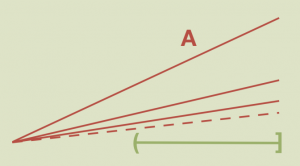# Aperiodvent, Day 7: CounterexamplesPictured here is a connected but not locally-connected space, from the endlessly counter-intuitive blog, Math Counterexamples. It’s full of counterexamples that will challenge your preconceptions about everything from geometry to algebra to Fubini’s theorem.

This is part of the Aperiodical Advent Calendar. We’ll be posting a new surprise for you each morning until Christmas!

## About the author

•#### Christian Lawson-Perfect

Mathematician, koala fan, Aperiodical editor. Usually found paddling in the North Sea, or fiddling with computers.

### 2 Responses to “Aperiodvent, Day 7: Counterexamples”

1.Joshua

Note: I know this is bad form, but I couldn’t figure out how to comment on the original author’s site. Also, I know that this point doesn’t directly relate to the headline attributes of the example, anyway…

The argument given that this space is not path connected doesn’t look right (though it is true the space isn’t path connected). They assume a path $\gamma(t)$ and then consider $\arg \gamma(t)$ the angle made by the vector $\vect{\gamma(t)}$ and the X-axis. This is supposed to be a continuous function. However, it isn’t defined for $t$ when $\gamma(t) = (0,0).$

I’m sure I’m missing something obvious, but don’t see it and am too tired right now to find an alternative argument (sic). At least I do know another space with the desired properties (and how to prove them), so I won’t be caught out in an emergency, while waiting for help with this one.

2.Josh
Well, I realized that there is a fix that is pretty easy. We want to show that paths from (3/4, 0) to (1,1) go through the origin. In fact, any path from (3/4, 0) to $(x, y)$ with $y>0$ must go through the origin. If such a path doesn’t, then we can restrict to the subspace excluding the origin. On that subspace, $\arg \gamma(t)$ is continuous, but the image has to be connected and the terminal value is an isolated point of the image. That is a contradiction, so the path must go through (0,0). Now, we can just look at the path from (3/4, 0) to (0,0).
Base on the previous argument, any such path must go to (0,0) before it can go to any point $(x, y)$ with $y>0$. That means we can restrict to just the subspace along the $x$-axis. However, that subspace isn’t even connected, let alone path connected, so we’re done.